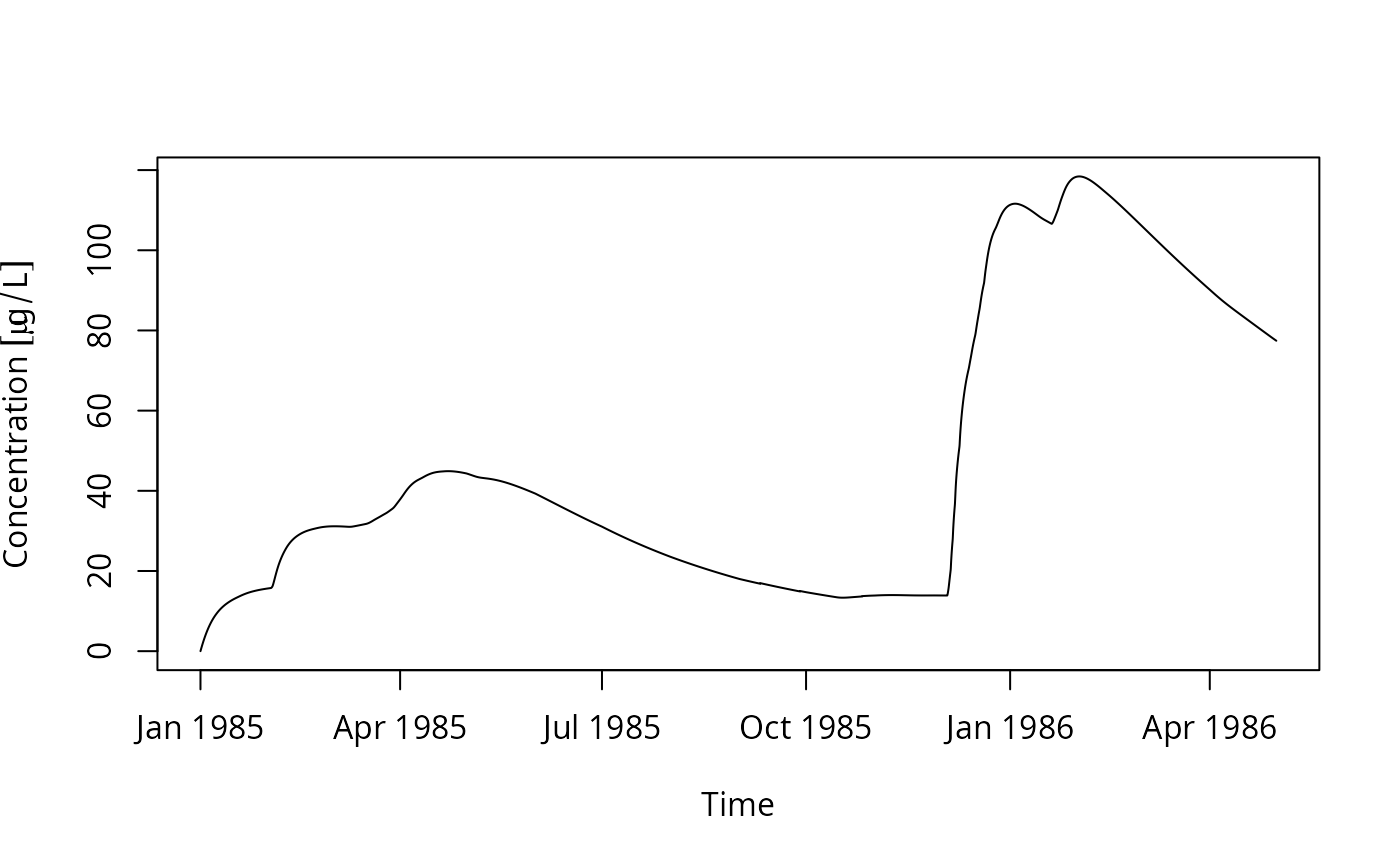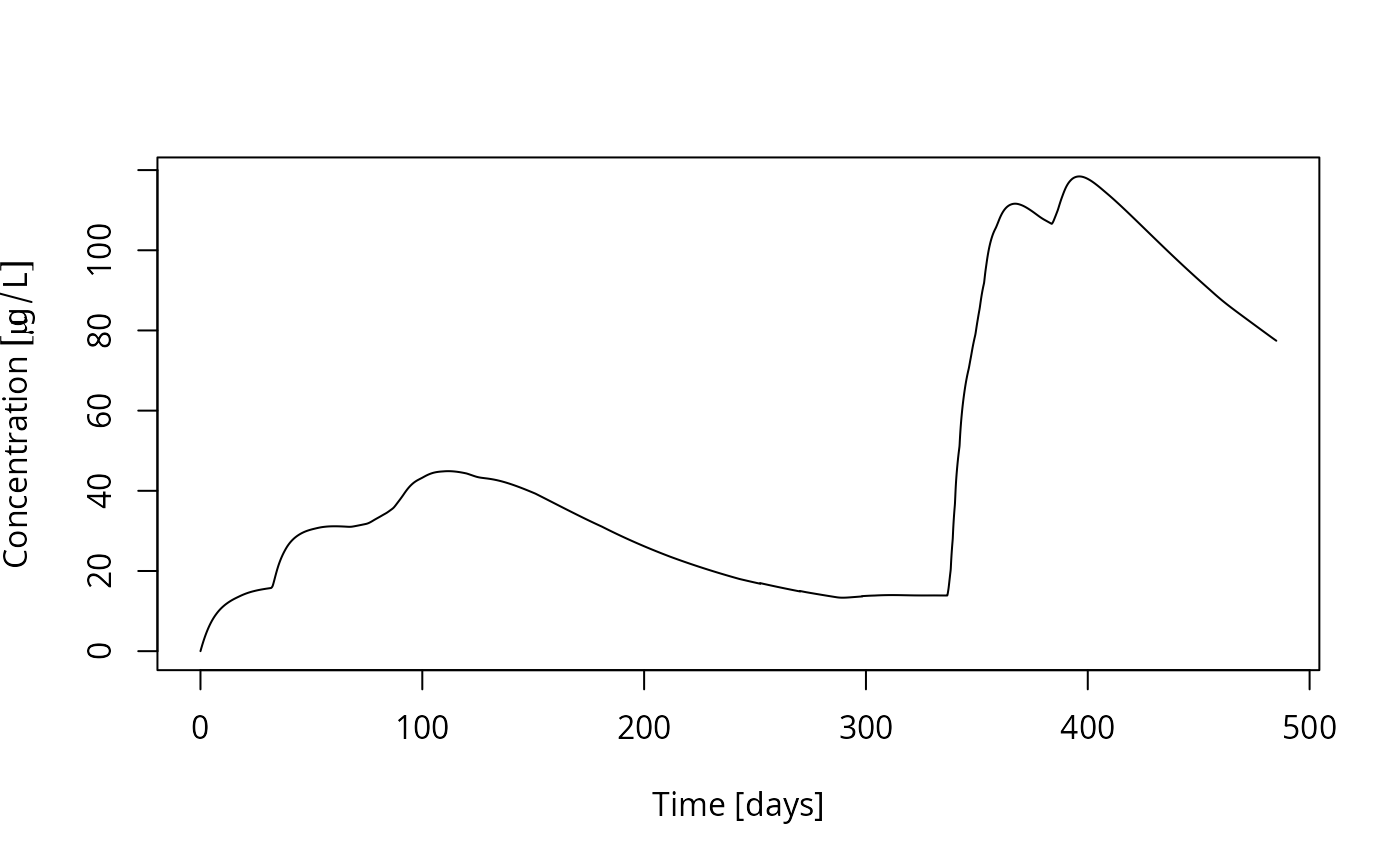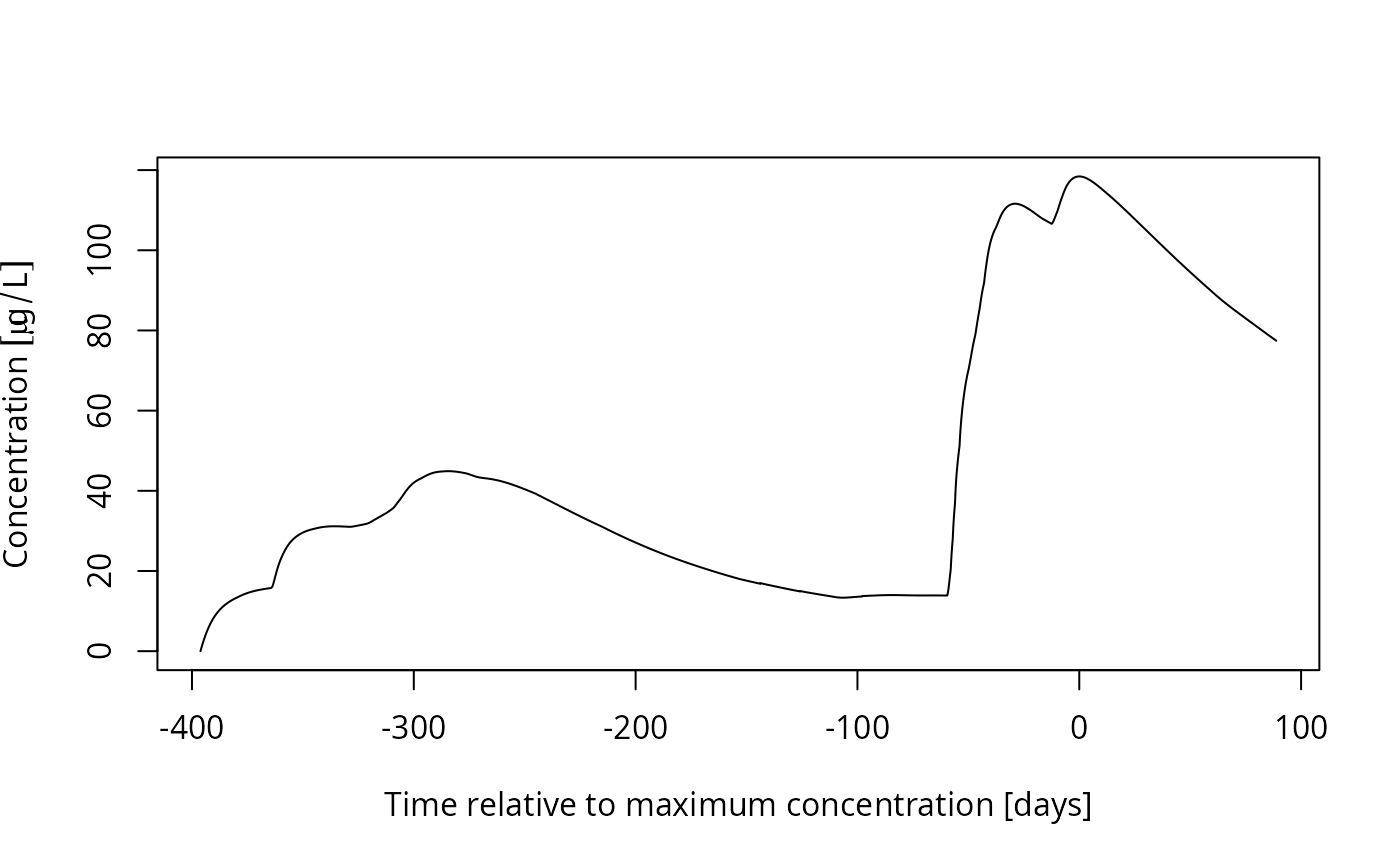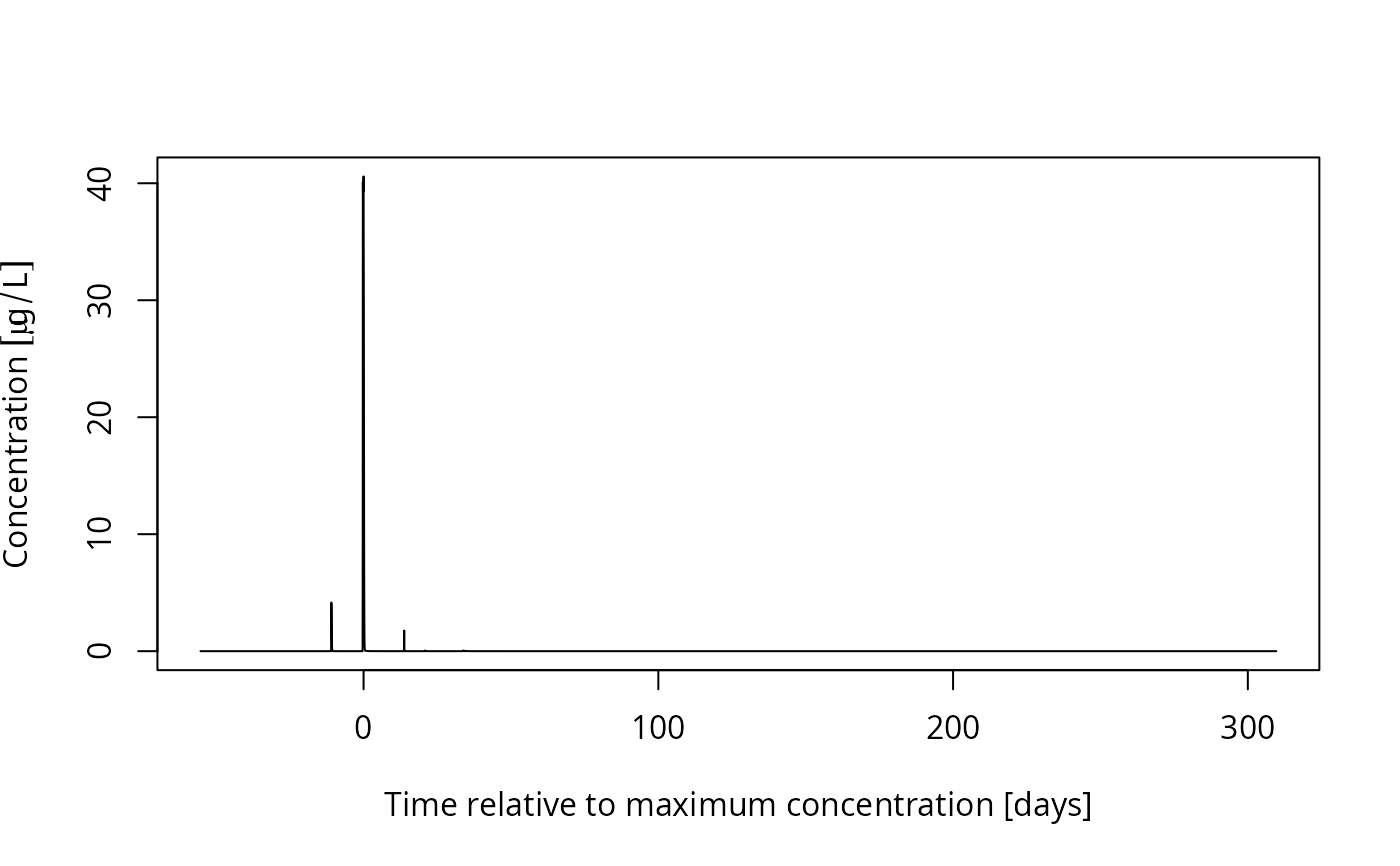Plot TOXSWA hourly concentrations of a chemical substance in a specific segment of a TOXSWA surface water body.

# S3 method for TOXSWA_cwa
plot(
x,
time_column = c("datetime", "t", "t_firstjan", "t_rel_to_max"),
xlab = "default",
ylab = "default",
threshold_factor = 1000,
thin_low = 1,
total = FALSE,
LC_TIME = "C",
...
)

## Arguments

x The TOXSWA_cwa object to be plotted. What should be used for the time axis. If "t_firstjan" is chosen, the time is given in days relative to the first of January in the first year. Labels for x and y axis. Should we add to an existing plot? The factor by which the data have to be lower than the maximum in order to get thinned for plotting (see next argument). If an integer greater than 1, the data close to zero (smaller than 1/threshold_factor of the maximum) in the series will be thinned by this factor in order to decrease the amount of data that is included in the plots Should the total concentration in water be plotted, including substance sorbed to suspended matter? Specification of the locale used to format dates Further arguments passed to plot if we are not adding to an existing plot

## Examples

H_sw_D4_pond  <- read.TOXSWA_cwa("00001p_pa.cwa",
basedir = "SwashProjects/project_H_sw/TOXSWA",
zipfile = system.file("testdata/SwashProjects.zip", package = "pfm"))
plot(H_sw_D4_pond)plot(H_sw_D4_pond, time_column = "t")plot(H_sw_D4_pond, time_column = "t_firstjan")plot(H_sw_D4_pond, time_column = "t_rel_to_max")plot(H_sw_R1_stream, time_column = "t_rel_to_max")